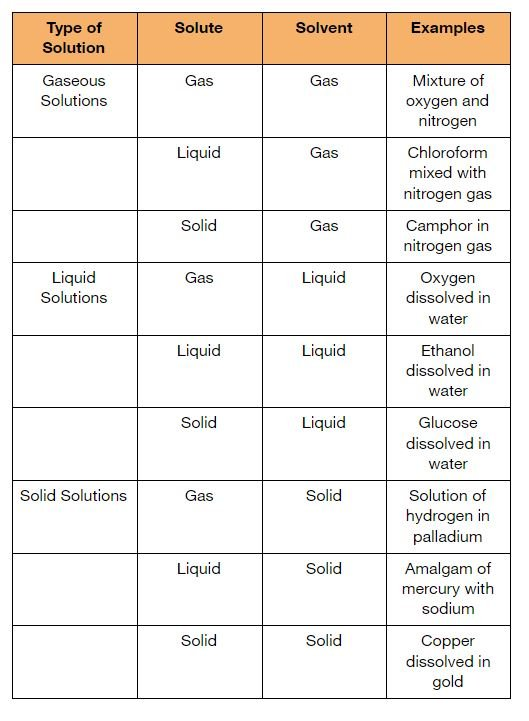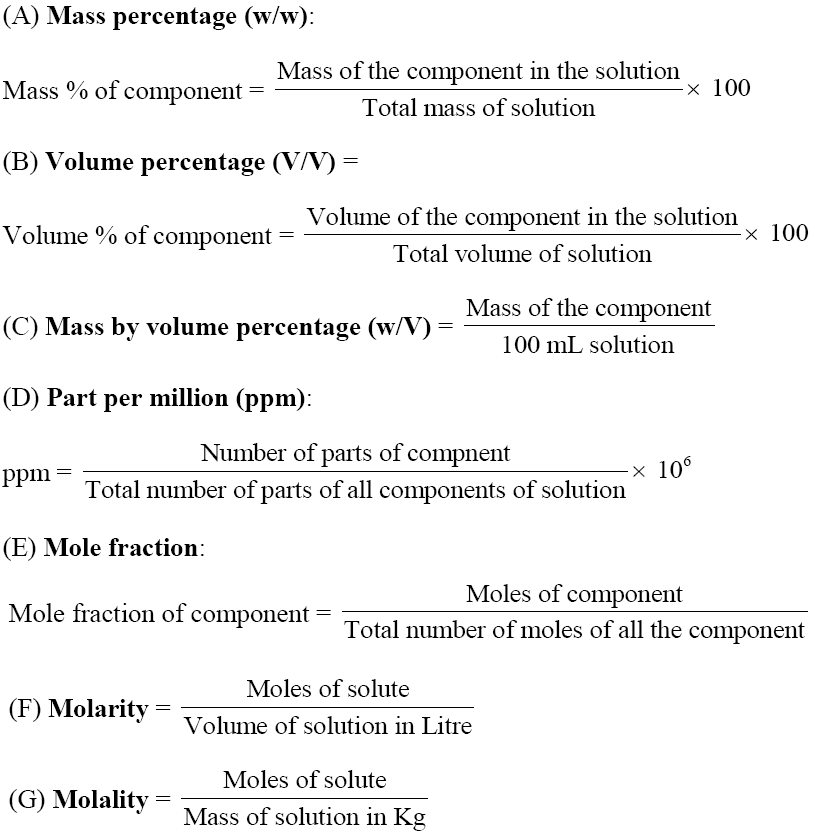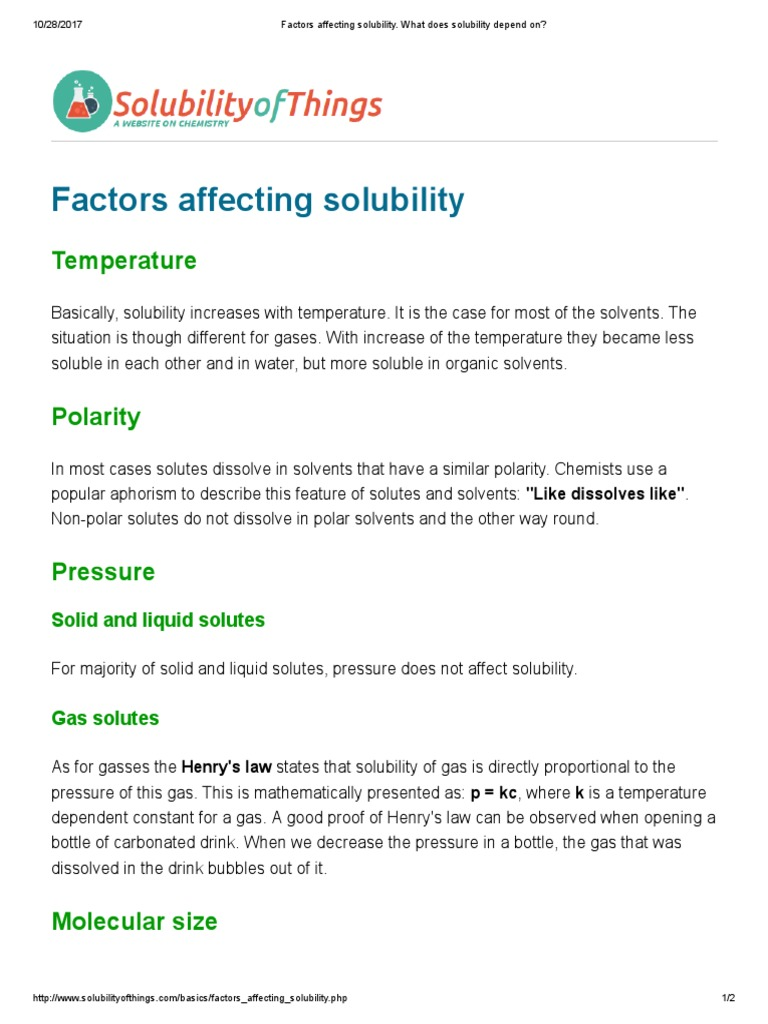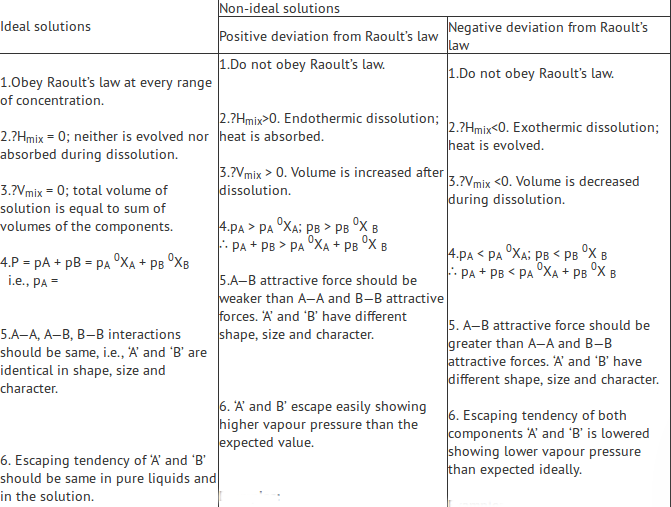# CBSE Class 12 Chemistry Chapter 2 Revision Notes

Chapter 2: Solutions Revision Notes

Solution : A perfectly homogeneous mixture of two or more components

Solute : The component which is present in lesser amount or whose physical state is changed during the formation of solution

Solvent : The component which is present in larger amount and determines the physical state of the solution

## Types of Solutions

• The three states of matter – solids, liquids and gasses may behave either as solute or solvent.
• Depending on the nature of the solvent, solutions are categorized as follows:Source

## Expressing Concentration of Solution

• Concentration of solute: The amount of solute dissolved per unit volume of solution.
• Strength of a solution is expressed in g/liter.Source

## Solubility

• Solubility : Maximum amount of substance that can be dissolved in a specified amount of solvent at a specified temperatureSource

Raoult’s law : It states that for a solution of volatile liquids, the partial vapour pressure of each component of the solution is directly proportional to its mole fraction present in solution. p1 = p°1 x1 and p2 = p°2 x2 where p°1 and p°2 are vapour pressures of pure components 1 and 2 respectively, at the same temperature.

Dalton’s law of partial pressures :

If y1 and y2 are the mole fractions of the components 1 and 2 respectively in the vapour phase then, p1 = y1 Ptotal and p2 = y2 Ptotal

Raoult’s law for solid-liquid solutions : It states that relative lowering in vapour pressure of a solution containing a non-volatile solute is equal to the mole fraction of the solute.

p° = vapour pressure of pure solvent

ps = vapour pressure of solution

x2 = mole fraction of solute.

## Ideal and Non-Ideal SolutionsSource

## Colligative Properties

Colligative properties : Properties which depend only on the number of solute particles dissolved in a definite amount of the solvent and not on the nature of the solute are called colligative properties.

Relative lowering of vapor pressure : When a nonvolatile solute is dissolved in a solvent, vapor pressure of the solution is lower than that of the pure solvent which is known as lowering of vapor pressure. Relative lowering of vapor pressure is equal to the mole fraction of the solute in the solution.

Elevation in boiling point : The boiling point of a solution containing a non-volatile solute is always higher than the boiling point of the pure solvent. This increase in boiling point is termed as elevation in boiling point.

Depression in freezing point : The freezing point of a solution containing a non-volatile solute is always less than the freezing point of the pure solvent. This decrease in freezing point is termed as depression in freezing point.

Osmosis and osmotic pressure : The movement of solvent molecules from less concentrated solution to more concentrated solution through a semipermeable membrane is termed as osmosis. The hydrostatic pressure which develops on account of osmosis is called osmotic pressure or the excess pressure that must be applied on the solution to prevent osmosis is called osmotic pressure.

Abnormal molecular mass : When the molecular mass of a substance determined by any of the colligative properties comes out to be different than the expected value, the substance is said to show abnormal molecular mass.

Van’t Hoff Factor : It is defined as the ratio of the experimental value of the colligative property to the calculated value of the colligative property.

]]>